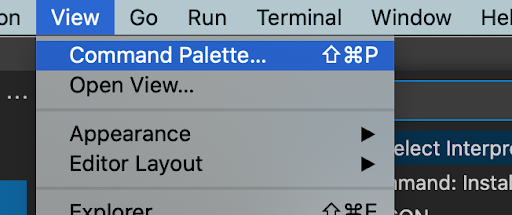Friday, December 21, 2018

Parsing a text file and importing values to MS Excel

We can use Excel VBA script to analyze a text file for a specific purpose. In this example, I have a text file that contains a list of files and their details like name, date created, file size, etc. This is an extraction of DIR command in the dos prompt. I am trying to find a text string "CNT" in each line and if it is present then I will extract the name of the file and store that in the Excel Cell along with the date the is created.

I have hardcoded the column here, you can modify it however you want. The first column will keep the name of the file and the second column will keep the file creation date.

Sub import_text_file_to_excel()
Dim fileHandle As Integer
Dim fileLine As String

Dim r As Integer
Dim c As Integer
r = 1
c = 1

Open "C:\\Users\\krsanjee\\files.txt" For Input As #1

While Not EOF(1)
Line Input #1, myline

On Error Resume Next
line_first_part = Left(myline, 40)
line_second_part = Right(myline, Len(myline) - 40)
If InStr(line_second_part, "CNT") Then
arr = Split(line_first_part, " ")
ActiveSheet.Cells(r, c).Value = arr(0)
ActiveSheet.Cells(r, c + 1).Value = line_second_part
r = r + 1
End If
Wend
Close #1
End Sub